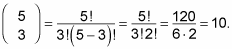##### Pre-Calculus Workbook For Dummies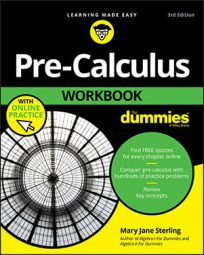Depending on how many times you must multiply the same binomial — a value also known as an exponent — the binomial coefficients for that particular exponent are always the same. The binomial coefficients are found by using the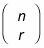combinations formula. If the exponent is relatively small, you can use a shortcut called Pascal's triangle to find these coefficients. If not, you can always rely on algebra!

Pascal's triangle, named after the famous mathematician Blaise Pascal, names the binomial coefficients for the binomial expansion. It is especially useful when raising a binomial to lower degrees.

For example, if a sadistic teacher asked you to find (3x + 4)10, you probably wouldn't want to use Pascal's triangle; instead, you'd just use the algebraic formula described shortly. The figure illustrates this concept. The top number of the triangle is 1, as well as all the numbers on the outer sides. To get any term in the triangle, you find the sum of the two numbers above it.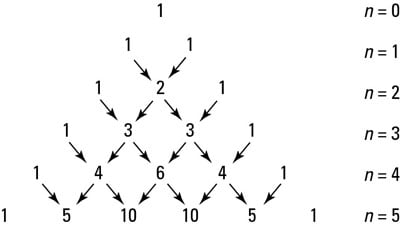Determining coefficients with Pascal's triangle

Each row gives the coefficients to (a + b)n, starting with n = 0. To find the binomial coefficients for (a + b)n, use the nth row and always start with the beginning. For instance, the binomial coefficients for (a + b)5 are 1, 5, 10, 10, 5, and 1 — in that order.

If you need to find the coefficients of binomials algebraically, there is a formula for that as well. The rth coefficient for the nth binomial expansion is written in the following form: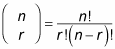You may recall the term factorial from your earlier math classes. If not, here is a reminder: n!, which reads as "n factorial," is defined as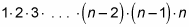You read the expression for the binomial coefficientas "n choose r." You usually can find a button for combinations on a calculator. If not, you can use the factorial button and do each part separately.

To make things a little easier, 0! is defined as 1. Therefore, you have these equalities: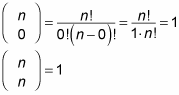For example, to find the binomial coefficient given by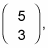substitute the values into the formula: